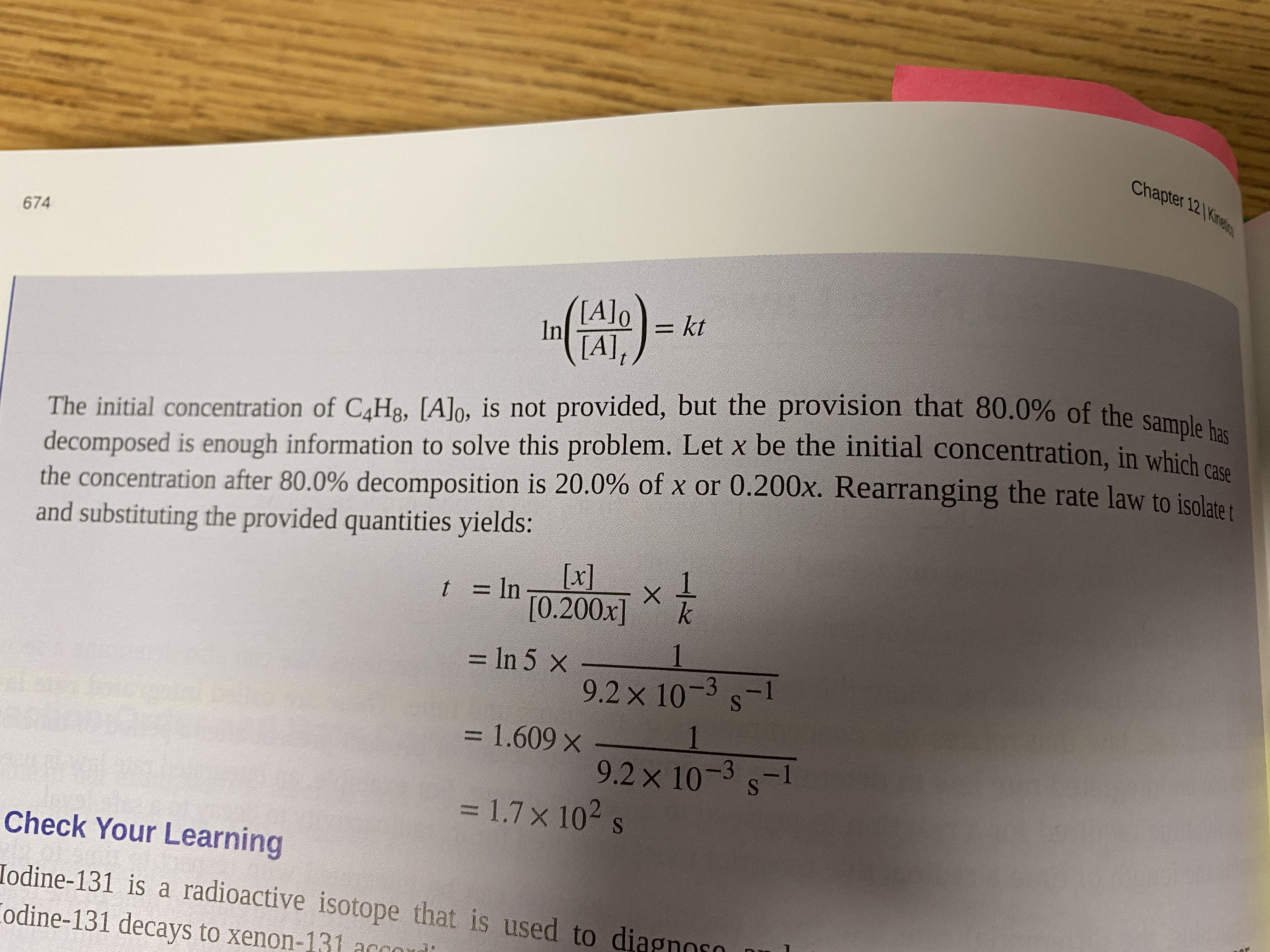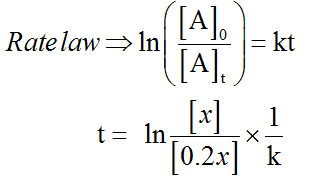# Chapter 12 Kine674()[A]o= kt%3DIn[A],The initial concentration of C4H3, [A]o, is not provided, but the provision that 80.0% of the sample hdecomposed is enough information to solve this problem. Let x be the initial concentration. in which ethe concentration after 80.0% decomposition is 20.0% of x or 0.200x. Rearranging the rate law to isolata .and substituting the provided quantities yields:[x][0.200x]t = ln%3D= In 5 x1%3D9.2x 10-3 s-1= 1.609 x1%D9.2 x 10-3 s-1= 1.7x 102 s%3DCheck Your LearningIodine-131 is a radioactive isotope that is used to diaCodine-131 decays to xenon-131

Question
31 views

I am not sure how they calculated the ln 5. I would like to possibly see a more detailed step for this solution.help_outlineImage TranscriptioncloseChapter 12 Kine 674 () [A]o = kt %3D In [A], The initial concentration of C4H3, [A]o, is not provided, but the provision that 80.0% of the sample h decomposed is enough information to solve this problem. Let x be the initial concentration. in which e the concentration after 80.0% decomposition is 20.0% of x or 0.200x. Rearranging the rate law to isolata . and substituting the provided quantities yields: [x] [0.200x] t = ln %3D = In 5 x 1 %3D 9.2x 10-3 s-1 = 1.609 x 1 %D 9.2 x 10-3 s-1 = 1.7x 102 s %3D Check Your Learning Iodine-131 is a radioactive isotope that is used to dia Codine-131 decays to xenon-131 fullscreen
check_circle

Step 1

The initial concentration, which 100% of C4H8 is not known. Let it be x.

About 80% of C4H8 is decomposed.

The final concentration of C4H8 at time t is 100% of x- 80% of x = 20% of x

That is 20/100(x) = 0.2x present at time t....

### Want to see the full answer?

See Solution

#### Want to see this answer and more?

Solutions are written by subject experts who are available 24/7. Questions are typically answered within 1 hour.*

See Solution
*Response times may vary by subject and question.
Tagged in

### Chemistry